# Not All Who Wander Are Lost

A Quick Start Guide to Multi Armed Bandits

Decisions are hard, they have always been. And when you finally find something you like, there is always that thought, in the back of your head - “can I find something better?”.

One of the challenges that arise in Reinforcement Learning is the Exploration - Exploitation Dilemma, do we choose - exploitation, where we make the best decision given current knowledge or exploration, where we gather more knowledge that might lead us to better decisions in the future.

In this post we will introduce the Multi Armed Bandits (MAB) problem, show two algorithms that solve this problem, and run a simulation.

# Multi Armed Bandits

The MAB problem, or bandit problem for short, is one of the simplest instances of the sequential decision making problem, in which a learner needs to select options from a given set of alternatives repeatedly in an online manner. The name comes from the gambling world in which a gambler decides from a row of slot machines, sometimes known as “one-armed bandits”.

# Algorithms

Before we get to the good stuff we need to understand what we are trying to achieve. As described, the MAB problem is a sequential decision making problem.

The “game” is set like this: in each round the agent decides on an action or arm x from a final set X, pulls the arm and receives a reward.

Each arm’s reward is associated with a probability distribution over [0, 1], each with an expectation μ. We will assume the existence of a unique best arm: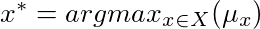To sum things up: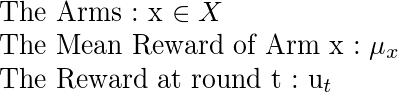The object of the game is to minimize the cumulative regret, that is defined as following:The cumulative regret shows the difference between the reward the player could have acquired if he played the best arm and the sum of rewards actually acquired.

Lets start with the naive case - assuming we have K arms, and T rounds to play, and we decide to play each arm T/K times - The regret that we would endure is linear with T. But we can do better!

## UCB

The most commonly used algorithm for the MAB setting ,the UCB algorithm, is used to minimize the cumulative regret. In each round the agent picks the arm with the highest “Upper Confidence Bound” (hence the name):The left part is the empirical estimation of the reward and the right part is the upper bound (β acts as a regulator and can be set to 1). The bound for each arm decreases as the number of times it had been picked so far grows. At each round, the chosen arm is the arm that potentially has the highest reward, either because it hadn’t been chosen enough, or because the arm’s average reward has been promising.

The UCB algorithm guarantees a logarithmic regret with T and is implemented like this: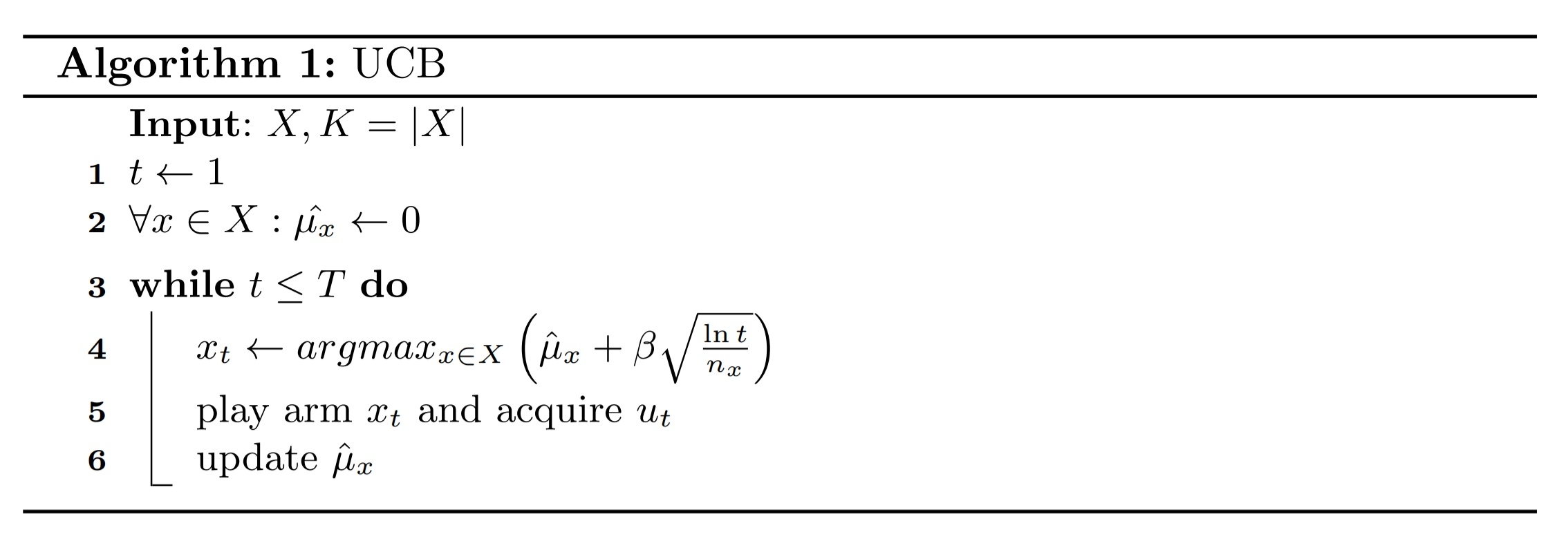## Thompson Sampling

The basic idea is to choose an arm to play according to its probability of being the best arm. The Thompson Sampling algorithm proceeds as follows. The algorithm maintains the number of successes and failures for each arm, and holds a random variable with a Beta distribution for each arm: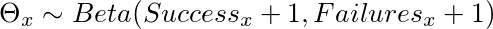At each round all the random variables of each arm are sampled. The arm with the highest corresponding value is chosen: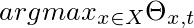The Thompson Sampling algorithm guarantees a logarithmic regret with T and is implemented like this: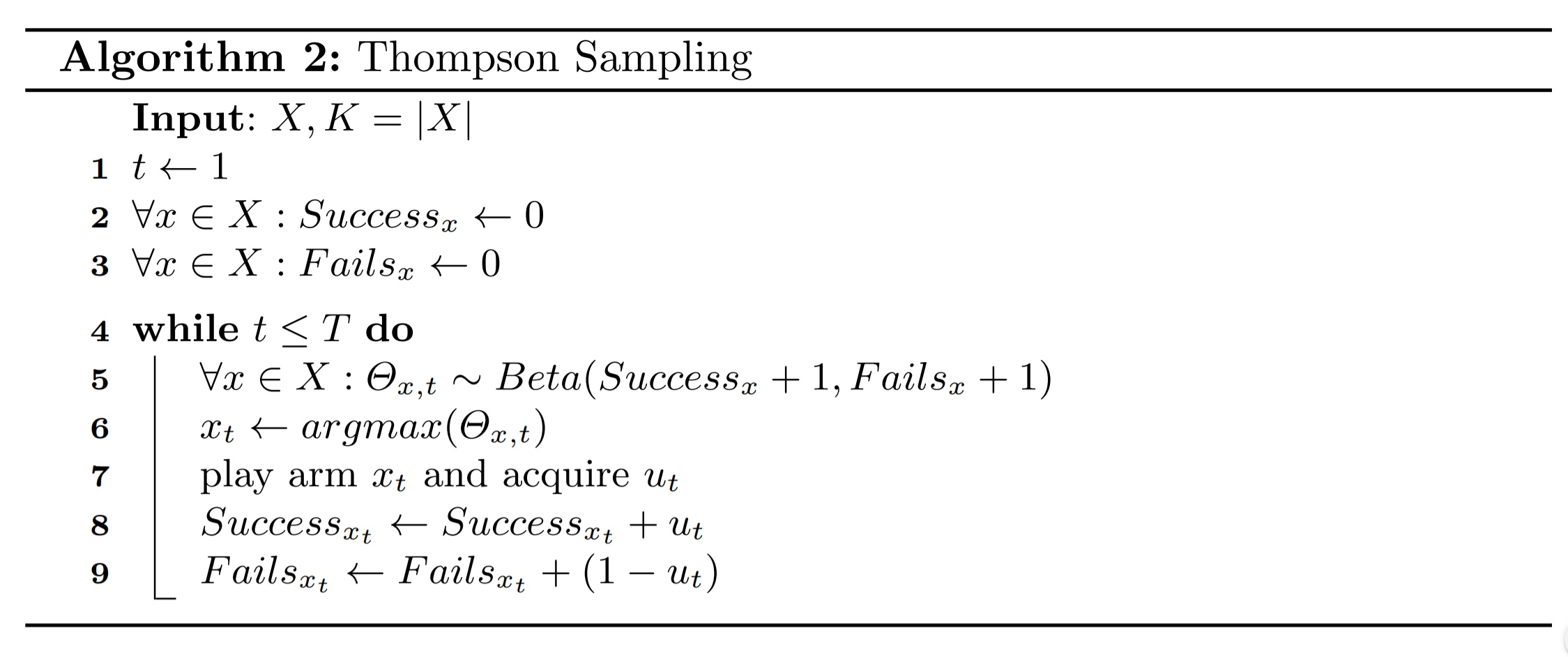# Implementation

In order to simulate a game we will need to implement the MAB algorithms and the arms.

The following classes hold the implementation of the UCB and Thompson Sampling algorithms.

Each class holds two methods:

• Select Arm - which selects the relevant arm according to the algorithm.
• Update - which updates the relevant values for the chosen arm.

We will use a Brnoulli Arm in this simulation, which draws a reward of either 0 or 1 according to the given mean:

# Simulation

In order to compare between the algorithms we will run a simulation that is defined by the number of arms, where the mean reward of each arm is set randomly, the number of rounds for each run, and the number of runs:

After running 500 games and collecting the data the comparison is very straight forward, where the middle line is the mean cumulative regret, and the upper and lower bounds are a single standard deviation from the mean.

# Conclusion

In this post we have defined the Multi Armed Bandit problem, showed two solutions and compared between them using a simulation.

In the next post we will show several other solutions that can handle different environments, such as partial information about the reward.